# Coefficient of restitution

Jump to: navigation, search

## Definition

QUICK PHRASES: bounciness of a collision, final velocity difference divided by initial velocity difference

### For a collision in one dimension

We first consider the case of a head-on collision, which can be considered a collision in one dimension.

Suppose two objects are moving along a one-dimensional axis, collide, and then again move along the same one-dimensional axis. If their initial velocities are (as signed real numbers)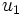$u_1$ and$u_2$ respectively and their final velocities are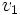$v_1$ and$v_2$ respectively, then the coefficient of restitution (abbreviated COR) or bounciness of the collision is defined as: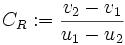$C_R := \frac{v_2 - v_1}{u_1 - u_2}$.

The coefficient of restitution is also sometimes denoted as$e$.

Note that the ordering of speeds in the numerator is opposite the ordering in the denominator. This reflects the fact that after the collisions, the direction of relative speed changes, because of a bouncing back effect.

### For a collision with a stationary object

If the collision is between a moving object and a heavy stationary object (which remains stationary even after the collision), then the coefficient of restitution is the ratio, with a negative sign, of the final velocity of the moving object to its initial speed. If the initial speed is$u$ and the final speed is$v$, the coefficient of restitution is given by: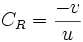$C_R = \frac{-v}{u}$.

Note that if an object remains stationary even after the collision, then it must be externally fixed. However, the stationary object approximation is valid when one of the objects is so much heavier than the other one that the velocity it acquires due to the collision is negligible.

### For a collision in three dimensions

For a collision in three dimensions, the velocities are vectors rather than scalars, so the above formula does not make sense. In this case, we interpret$u_1,u_2,v_1,v_2$ as the components of velocities in the normal direction, i.e., the direction perpendicular to the plane of contact/tangent plane at the point of contact.

## Constitutive factors

The coefficient of restitution is dependent on the specific collision and cannot be inferred precisely from the geometry, velocity, and the kind of material in the colliding bodies. However, knowledge of the type of material and the geometry of the bodies usually allows for an approximate estimation of the coefficient of restitution.

## Particular cases

Type of collision Value of coefficient of restitution What else this means
Perfectly elastic collision 1 both linear momentum and kinetic energy are preserved
Perfectly inelastic collision 0 the two bodies merge into one. Linear momentum is preserved in a manner that causes the maximum possible loss in kinetic energy.
Inelastic collision that is not perfectly inelastic between 0 and 1 the bodies do not merge. Linear momentum is preserved and some kinetic energy is lost.
Superelastic collision greater than 1 there is a gain in kinetic energy

## Experimental measurement

Experimentally measured construct Relationship with the coefficient of restitution
The fraction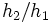$h_2/h_1$ where$h_1$ is the original height from which a ball is dropped and$h_2$ is the height to which it rebounds This is the square of the coefficient of restitution, because of the relation$v^2 = 2gh$ between velocity at ground level and maximum height attainable.